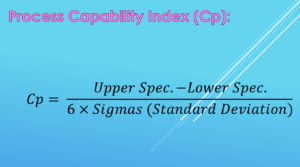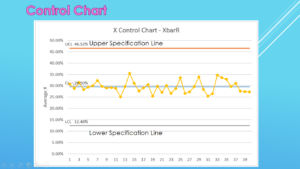Achieve Innovative Process Improvement＋Standardization by IT System with MS 365.# Process Capability Basics, Cp and Cpk Deference and Unilateral Tolerance\ この記事を共有 /
Process Capability Basics, Cp and Cp...

The calculation for the Process Capability Index is “Your process’s CTQ Specification Interval divided by 6 Sigmas”. It is divided into ‘CTQ’, ‘Specification Interval’ and ‘6 Sigmas’ and each is illustrated to be easily understood.

(Duration: 7:17)

＜＜ Related Posts ＞＞

## Today, we’ll discuss the basics of Process Capability, Cp, Cpk and Unilateral (one-sided) Tolerance.

Hi, this is Mike Negami, Lean Sigma Black Belt. Recently, I received a question from Paul, one of my viewers.

“Need to understand statistical method for unilateral tolerance.”

Thank you, Paul for your question. By the way, if you have a video request or any questions, please write them in the comment section below.

## Before Unilateral Tolerance, the Basics of Process Capability Index

This is a question of unilateral, or one-sided, tolerance, but before talking about that, we need to know the Process Capability Index. Generally, Cp and Cpk are used in the manufacturing industry.  Even if you’re in a service operation, you should learn these basic concepts.

Let me ask you something, how can you rate superiority and inferiority among several manufacturing lines in a factory?  We call this production capability “Process Capability”, and use a Process Capability Index as its quantification.

The calculation for the Process Capability Index is “Your process’s CTQ Specification Interval divided by 6 Sigmas”.  There are three words which you may not know well: ‘CTQ’, ‘Specification Interval’ and ‘6 Sigmas’.

‘CTQ’, which stands for ‘Critical To Quality’, is the most important factor that affects the quality of products on each line, for example: length, weight, resistance value etc.  I explained about CTQ in my previous post. Please click the link below and read the post.

＜＜ Related Post ＞＞

Regarding ‘Specification Interval’, in the manufacturing industry, they aim their CTQ measurements for a certain range during manufacturing.  The upper and lower lines of the range are Specifications. Specifications are decided by product designers or the industries. The subtraction of the specifications is ‘Specification Interval’.

The last one is ‘6 Sigmas’.  We can describe the variation of a numerical data group by standard deviation.  The standard deviation is statistically called Sigma. 6 Sigmas are exactly a value six times the standard deviation.  The Process Capability Index is called Cp.

## How to calculate the Process Capability Index, Cp

Let’s see that formula.Since the Specification Interval, which is a numerator, is decided ahead of time, it’s a constant. Therefore, what affects the value of Cp is only 6 Sigmas, which is the standard deviation of the measured data.

Since it’s a denominator, the greater the standard deviation, which is, the greater the variance of process, the smaller the value of Cp becomes, and the smaller the standard deviation, the larger the value of Cp. After all, it means that the greater the Process Capability Index, the better the factory line is. It’s actually a very simple concept.

However, there is one condition when using Cp: Your process has to be “under absolute control”.
“Under absolute control” means that when you see a control chart, all the plots are kept within the Specification lines, and additionally, the average of the plots is the center value of the Specification Interval range. Statistically it is said to follow the normal distribution.## How to calculate the Process Capability Index, Cp

In practice, you cannot use this Cp because the average of your data value and the center value of the Specification Interval are almost always off.  Instead there is Cpk that corrects the deviation. In fact, always use this one. The concepts behind the two are the same, but the calculation formula is somewhat different.

First, calculate the following two formulas: The Upper Specification minus average, then divide by 3 Sigmas and the average minus the Lower Specification, then divide by 3 Sigmas. Then, adopt the smaller one.

## Unilateral Tolerance’s Basics and Formula

Let’s see Paul’s detailed question on one-sided tolerance.

“Example: Finish of material 1.32 Maximum means that upper tolerance is 1.32 and lower tolerance is 0 (Zero). How do we validate the process by an using appropriate statistical method?”

In his example, anything less than 1.32 is acceptable. In that case, there is only an Upper Specification Limit and no Lower Specification Limit.

Actually we can use the exact same formula of Cpk. Set the Upper Specification Limit to 1.32 and the Lower Specification Limit to 0. Let’s do it here.

I prepared a set of data as measurement data here. The average value is 1.301 and standard deviation is 0.006. You can easily get those results with these Excel functions.Put them in the Cpk’s formula, then Cpk with the Upper Specification Limit is 1.056 and Cpk with the Lower Specification Limit is 72.278. Since you adopt smaller one, the

Process Capability Index Cpk in this example is 1.056. Generally, if your Ckp is more than 1.33, your process is considered good, but your company should set that standard first.

### Comment

1..edu says:

I know this website gives quality based content and additional information, is there any other site which
gives these things in quality?